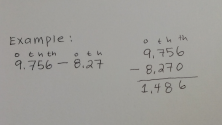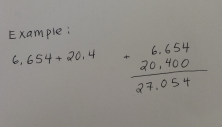Smartick is an advanced online program that teaches kids math and coding in only 15 min. a day
Try it for free!

Jul01

# Working with Decimals: Addition and Subtraction

In this post we are going to see some addition and subtraction calculations with decimals.

In order to add or subtract decimals, we need to stack the numbers that have a decimal point one on top of the other, making sure to line up the values in the same columns. By doing so, the tens, and even hundredths, places will be lined up.

Let’s look at some examples:We are going to subtract 8.27 from 9.756. So, we’ll have to line up the values. That’s to say, tens place under tens place, hundredths place under hundredths place, and so on, just like you can see in the picture.

The number 8.27 doesn’t have a number in the millionths place so we can put a 0 there and it’ll be easier for us to go on with the calculation. Now, we can move on to subtracting the numbers, making sure to write the decimal point in the same place. The final answer is 1.486.

Now, we have to add 6.654 and 20.4. Just like we saw in the prior example and in the picture above, we have to line all of the place values up—the tens, hundredths and thousandths places—so that they fall in the same column.The number 20.4 doesn’t have a hundredths or thousandths place, so we can put 0s in these spots to make the calculation simpler. Now, we can add the two numbers, making sure to maintain the decimal point in the same spot in the final answer. The final answer is 27.054

In addition to the post, you can find more information to practice with decimals in the following links.

And if you want to learn much more elementary math, sign up for Smartick today!

Learn More:

Latest posts by Smartick (see all)